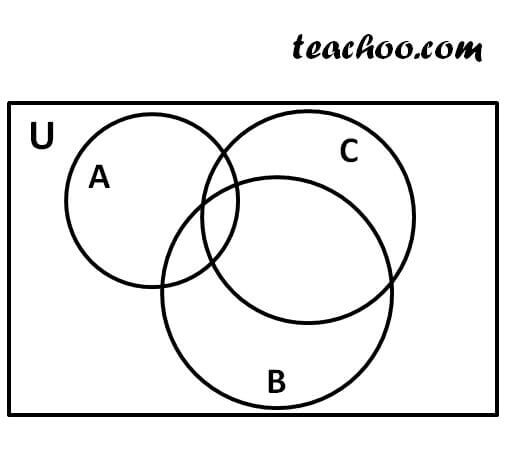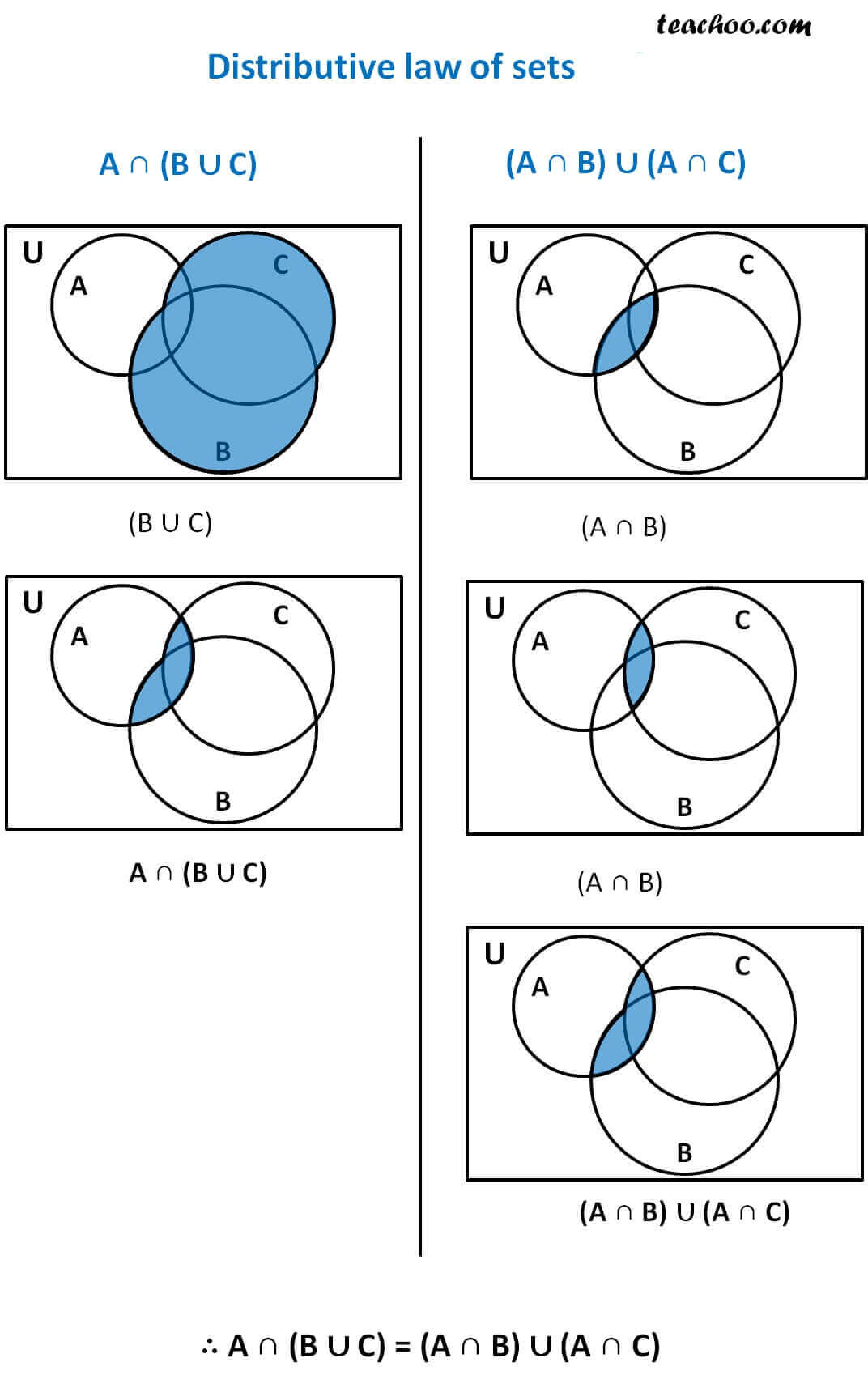Intersection of Sets

Chapter 1 Class 11 Sets
Concept wise

Distributive law of set is

A ∩ (B ∪ C) = (A ∩ B) ∪ (A ∩ C )

Let us prove it by Venn diagram

Let’s take 3 sets – A, B, CWe have to prove

A ∩ (B ∪ C) = (A ∩ B) ∪ (A ∩ C)Distributive law is also

A ∪ (B ∩ C) = (A ∪ B) ∩ (A ∪ C )

this can also be proved in the same way.

Proof using examples is done here

Get live Maths 1-on-1 Classs - Class 6 to 12